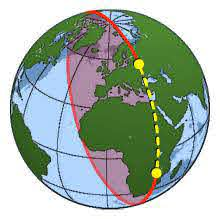# Non-Euclidean Geometry

Non-Euclidean geometry is any type of geometry that is different from the “flat” (Euclidean) geometry you learned in school. It’s a set of geometries where the rules and axioms you are used to get broken: parallel lines are no longer parallel, circles don’t exist, and triangles are made from curved lines.In spherical geometry, the shortest distance between two points isn’t a straight line: it’s a great circle.

## Why Do We Need “Other” Geometry?

Those proofs of high school geometry are based on a set of rules laid down in Euclid’s Elements. It’s the system of rules for geometry that were used for thousands of years. However, as the study of points, lines, surfaces and solids progressed, mathematicians began to realize that Euclid’s rules were wholly inadequate. For example, Euclid never mentioned that “…a circle has an inside and an outside and an inside, that triangles can be turned over, and other assumptions essential to his system” (Gardner, 1997).

## Types of Non-Euclidean Geometry

There are many different areas of Non-Euclidean geometry. Each has their own distinct set of rules:

• Elliptic Geometry: There are no parallel lines in this geometry, as any two lines intersect at a single point,
• Hyperbolic Geometry: A geometry of curved spaces. Played a vital role in Einstein’s development of relativity (Castellanos, 2007).
• Minkowski Geometry: Geometry in a finite number of dimensions (for example, the fourth dimension) where distances aren’t uniform in every direction. Famous for its use in Einstein’s special relativity.
• Projective Geometry: Deals with the relationships between geometric figures and how they project onto different surfaces,
• Spherical Geometry: plane geometry on the surface of a sphere. Like elliptic geometry, there are no parallel lines. Commonly used by explorers and navigators.
• Taxicab Geometry: Based on how a taxicab moves through the square grids of New York City streets, this branch of mathematics uses square grids to measure distances.

## References

Blackman. Albert Einstein and the Theory of Relativity. Retrieved November 16, 2020 from: http://www.pas.rochester.edu/~blackman/ast104/einstein.html Castellanos, J. (2007). 3: What is Non-Euclidean Geometry. Retrieved November 16, 2020 from: https://www.cs.unm.edu/~joel/NonEuclid/noneuclidean.html Coxeter, H. (1988). Non-Euclidean Geometry. Cambridge University Press. Gardner M. (1997) Non-Euclidean Geometry. In: The Last Recreations. Springer, New York, NY. https://doi.org/10.1007/978-0-387-30389-5_19

CITE THIS AS:
Stephanie Glen. "Non-Euclidean Geometry" From StatisticsHowTo.com: Elementary Statistics for the rest of us! https://www.statisticshowto.com/non-euclidean-geometry/